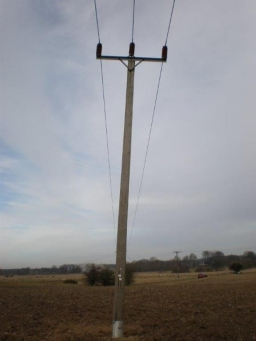# Seedlings 30831

Three workers planted 3,555 seedlings a day. The first worked in the standard. The second planted 120 more and the third 135 seedlings more than the first. How many seedlings did each worker plant?

a =  1100
b =  1220
c =  1235

### Step-by-step explanation:

a+b+c=3555
b=120+a
c=a+135

a+b+c = 3555
a-b = -120
a-c = -135

Row 2 - Row 1 → Row 2
a+b+c = 3555
-2b-c = -3675
a-c = -135

Row 3 - Row 1 → Row 3
a+b+c = 3555
-2b-c = -3675
-b-2c = -3690

Row 3 - -1/-2 · Row 2 → Row 3
a+b+c = 3555
-2b-c = -3675
-1.5c = -1852.5

c = -1852.5/-1.5 = 1235
b = -3675+c/-2 = -3675+1235/-2 = 1220
a = 3555-b-c = 3555-1220-1235 = 1100

a = 1100
b = 1220
c = 1235

Our linear equations calculator calculates it.Did you find an error or inaccuracy? Feel free to write us. Thank you!

Tips for related online calculators
Do you have a linear equation or system of equations and looking for its solution? Or do you have a quadratic equation?